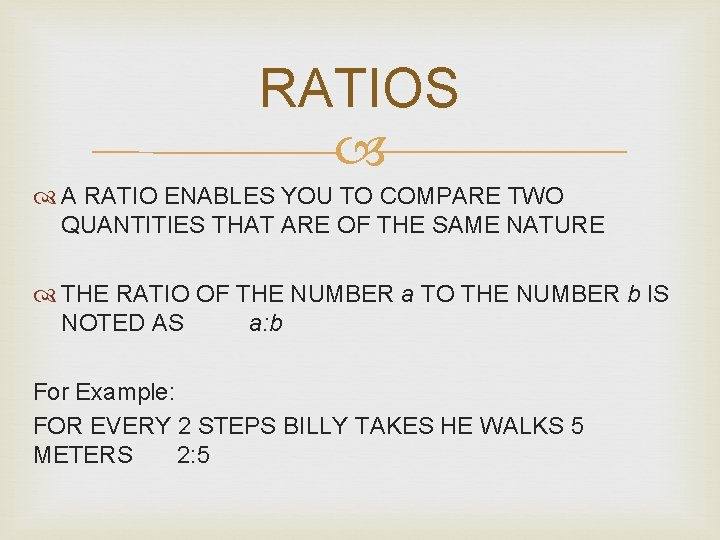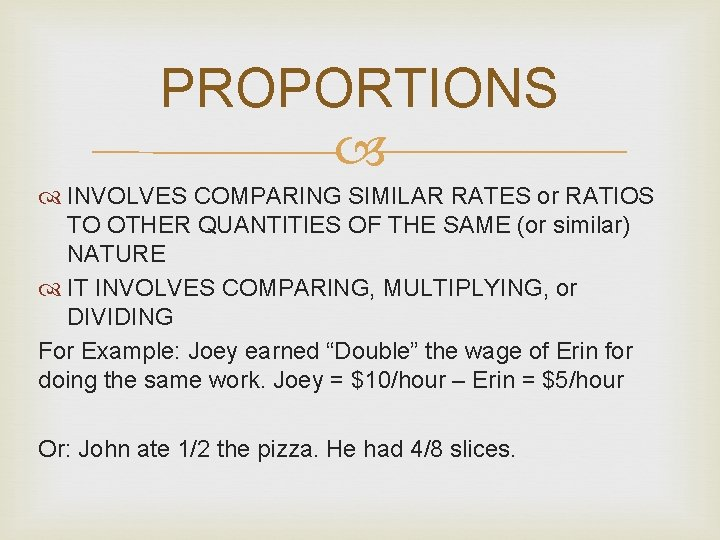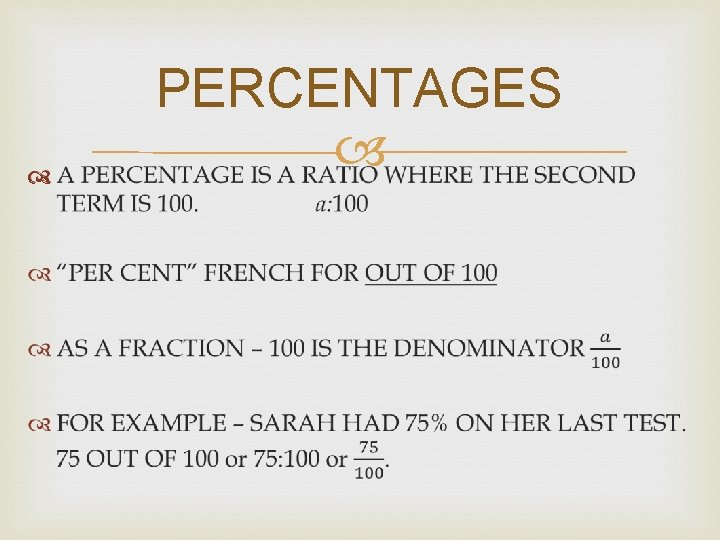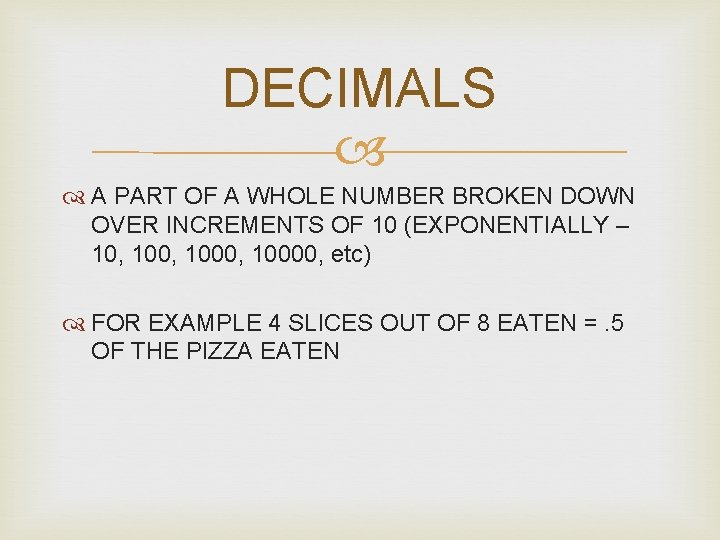# UNIT 2 RATES RATIOS PROPORTIONS RATES RATIOS PROPORTIONS

• Slides: 7UNIT 2 – RATES, RATIOS, & PROPORTIONS - RATES - RATIOS - PROPORTIONS - PERCENTAGES - FRACTIONS - DECIMALSRATE As in “UNIT RATE” A COMPARISON OF TWO QUANTITIES MEASURED IN DIFFERENT TYPES OF UNITS For Example: Peter earned \$27 for 3 hours worth of work. How much did he make per hour (Unit = money & Unit = hour) \$27 ÷ 3 h = \$9 per hour or 3 h ÷ \$27 =. 11 hours per dollar (18 min to earn \$1)RATIOS A RATIO ENABLES YOU TO COMPARE TWO QUANTITIES THAT ARE OF THE SAME NATURE THE RATIO OF THE NUMBER a TO THE NUMBER b IS NOTED AS a: b For Example: FOR EVERY 2 STEPS BILLY TAKES HE WALKS 5 METERS 2: 5PROPORTIONS INVOLVES COMPARING SIMILAR RATES or RATIOS TO OTHER QUANTITIES OF THE SAME (or similar) NATURE IT INVOLVES COMPARING, MULTIPLYING, or DIVIDING For Example: Joey earned “Double” the wage of Erin for doing the same work. Joey = \$10/hour – Erin = \$5/hour Or: John ate 1/2 the pizza. He had 4/8 slices.PERCENTAGESFRACTIONSDECIMALS A PART OF A WHOLE NUMBER BROKEN DOWN OVER INCREMENTS OF 10 (EXPONENTIALLY – 10, 1000, 10000, etc) FOR EXAMPLE 4 SLICES OUT OF 8 EATEN =. 5 OF THE PIZZA EATEN